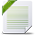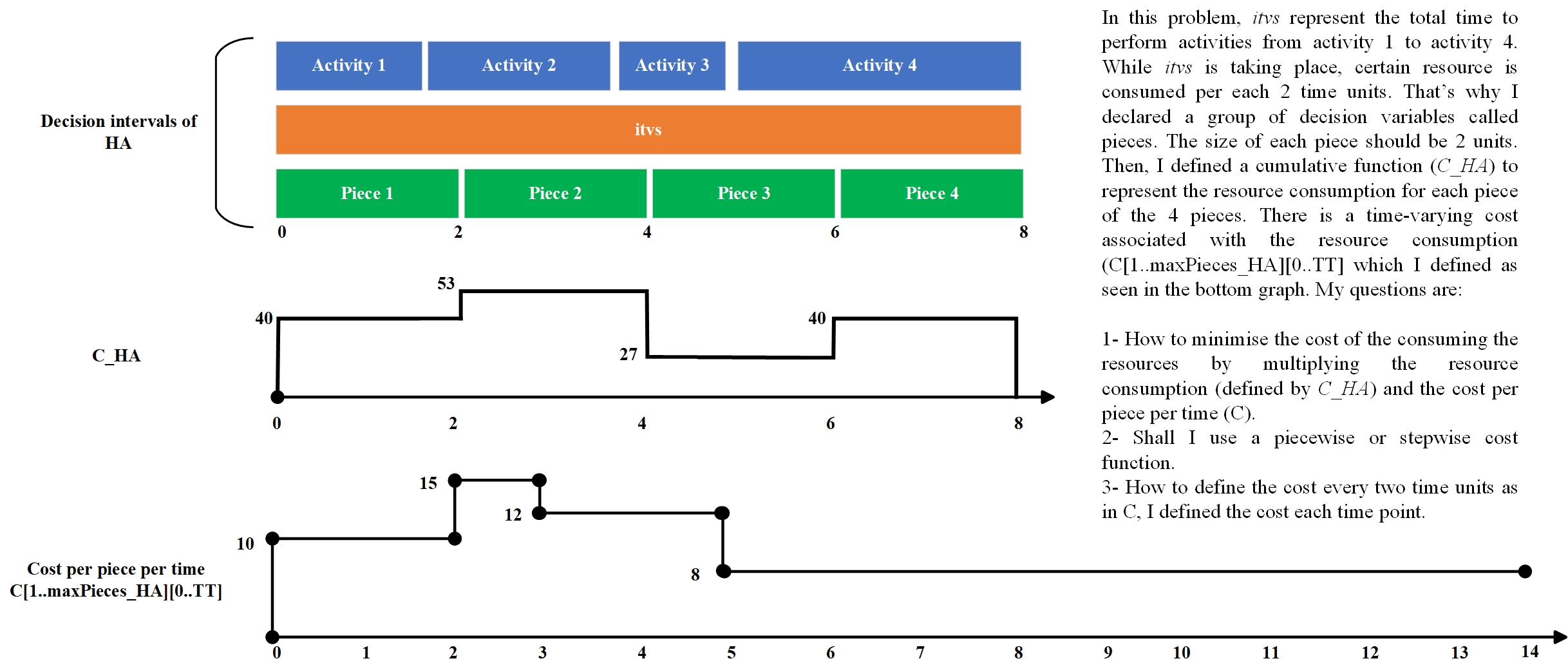# Decision Optimization

View Only

## Using piecewise/stepwise functions with decision variables to minimise cost#### Mohamed AwadTue July 27, 2021 03:48 PM#### Philippe RefaloThu July 29, 2021 12:35 PM#### Mohamed AwadThu July 29, 2021 03:14 PM#### ALEX FLEISCHERFri July 30, 2021 02:48 AM#### Mohamed AwadSun August 01, 2021 05:27 AM• #### 1.  Using piecewise/stepwise functions with decision variables to minimise cost

Posted Tue July 27, 2021 03:48 PM

Dear CP experts,

I would be grateful if you could help me with the following question. I have a scheduling problem at which I am trying to minimise its cost with respect to the time at which the scheduled activities occur. For simplicity, I translated my scheduling problem to a house building problem which is a common problem.

There are two Houses categories; HA and HB. Each house has a set of activities that needs to be done. Each activity has a duration, waiting times and a precedence activity. The makespan on each house is modelled by itvs while the assignment decision of certain managers ManNames is modelled by wtasks.

I added a set of optional decision variable for each house; pieces_HA and pieces_HB which are used to associate the cost of itvs by defining the cost per time for each segment of pieces_HA and pieces_HB. For simplicity, I focused on HA so, I defined a piecewise cost function to model the cost value when each segment of pieces_HA takes place. The amount of the required resource for each segment is modelled using the cumulative function C_HA.

What I would like to do is to minimise the following equation:

Total cost = amount of required resource X cost of the required resource based on the time

When I used the piecewise function, the values were logical until I replaced:

pwlFunction Cost[i in 1..maxPieces_HA] = piecewise(t in 0..TT-1) {C[i][t+1]-C[i][t]-> (t+1); 0}(0,C[i]);

by:

pwlFunction Cost[i in 1..maxPieces_HA] = piecewise(t in 0..TT-1) {C[i][t+1]-C[i][t]-> 2*(t+1); 0}(0,C[i]);

My question is how to implement the total cost equation, why the cost function became different after I multiplied it by 2 and whether the stepwise function would work better that the piecewise?

P.S. I attached the *.Mod and *.Dat files

Mohamed

The piecewise function I used on the model was adopted from the following link:

------------------------------
------------------------------

Attachment(s)OPL_E4.dat   1 KB 1 versionOPL_E4.mod   5 KB 1 version

• #### 2.  RE: Using piecewise/stepwise functions with decision variables to minimise cost

Posted Thu July 29, 2021 12:35 PM
Hi

Can you explain what do you mean by "amount of required resource" ? If it is the integral of the cumul function, it can be computed by summing the product of the interval length and cost :

sum(a in allActivities_2,j in 1..maxPieces_HA:a.Houses=="HA") lengthOf(pieces_HA[a][j], 0) * Cost_HA[j];

Can you be more precise about "cost of the required resource based on the time" ? I assume it is the piecewise linear function in your model.

Note that replacing

pwlFunction Cost[i in 1..maxPieces_HA] = piecewise(t in 0..TT-1) {C[i][t+1]-C[i][t]-> (t+1); 0}(0,C[i]);

by:

pwlFunction Cost[i in 1..maxPieces_HA] = piecewise(t in 0..TT-1) {C[i][t+1]-C[i][t]-> 2*(t+1); 0}(0,C[i]);

You multiply the position of the breakpoint by 2. Is that what you want ? Wouldn't it be rather the slope that you would like to multiply ?

A possible way to obtain what you want is to multiply the sum above by the piecewise linear function for each resource.

Note that itvs variable are always present and thus you have many warning of this type:

Warning: Boolean expression 'presenceOf' is always true because interval variable 'itvs({"HA",1})' is declared present.
presenceOf("itvs({\"HA\",1})")

Regards,

Philippe

------------------------------
Philippe Refalo
IBM ILOG CP Optimizer
------------------------------

• #### 3.  RE: Using piecewise/stepwise functions with decision variables to minimise cost

Posted Thu July 29, 2021 03:14 PM
Hi Philippe,

Thank you for your reply. I am attaching a schematic drawing describing the problem. I hope this makes it clear. Also, I am re-attaching the *.Mod and *.Dat after I changed some values to match the schematic problem.Mohamed

------------------------------
------------------------------

Attachment(s)OPL_E4.dat   1 KB 1 versionOPL_E4.mod   5 KB 1 version

• #### 4.  RE: Using piecewise/stepwise functions with decision variables to minimise cost

Posted Fri July 30, 2021 02:48 AM
Hi,

in how to with OPL
have you seen
How to describe a piecewise linear function with breakpoints instead of slopes ?

and

How to describe a stepwise function with breakpoints instead of jumps ?

``````     tuple breakpoint // y=f(x)
{
key float x;
float y;
}

sorted { breakpoint } breakpoints={<0,0>,<1,1>,<2,4>};

stepFunction f=stepwise(b in breakpoints:b!=first(breakpoints))
{ prev(breakpoints,b).y->b.x; last(breakpoints).y } ;

assert forall(b in breakpoints) f(b.x)==b.y;​``````

------------------------------
[Alex] [Fleischer]
[EMEA CPLEX Optimization Technical Sales]
[IBM]
------------------------------

• #### 5.  RE: Using piecewise/stepwise functions with decision variables to minimise cost

Posted Sun August 01, 2021 05:27 AM
Hi Alex,

Thank you for your reply. Actually, I am a little bit confused about the difference between the piecewise and stepwise functions. I know that the stepwise is a special case of the piecewise function when the slope is zero but  I don't know how to use the stepwise with a decision variable. I know that the piecewise can be used in objective function expressions using startEval and endEval for example. Is there a way to use the stepwise similarly?

Also, when I multiplied the breakpoint by 2 in my example, the objective function became 22 however, if I don't do that, the objective function became 31. Could you please advise on how the objective function value was calculated for each case.

Mohamed

------------------------------
------------------------------

• #### 6.  RE: Using piecewise/stepwise functions with decision variables to minimise cost

Posted Mon August 02, 2021 10:56 AM

Your example help but still the solution is not that clear : why is there a drop in the cost at time 3 from 15 to 12 ? This seems unrelated to the activity positions above.

For item 1, you haven't said how you want the resource consumption to appear in the objective function. You can use the integral of the cumul function over a range, or the maximum for instance. Note that a cumul expression can be constrained with ==, <= or >= constraints. You cannot turn it into a numerical value unless you use, for instance, the computation I presented in my precedent post.

Your function is a stepwise function as far as we can see.

If you want the pieces activity to start at even values, you can add a constraint like start(piece) = 2 * additional_variable where additional_variable is an integer decision variable with the appropriate range or you can add the constraint startOf(piece) mod 2 == 0;

Regards

Philippe

------------------------------
Philippe Refalo
IBM ILOG CP Optimizer
------------------------------